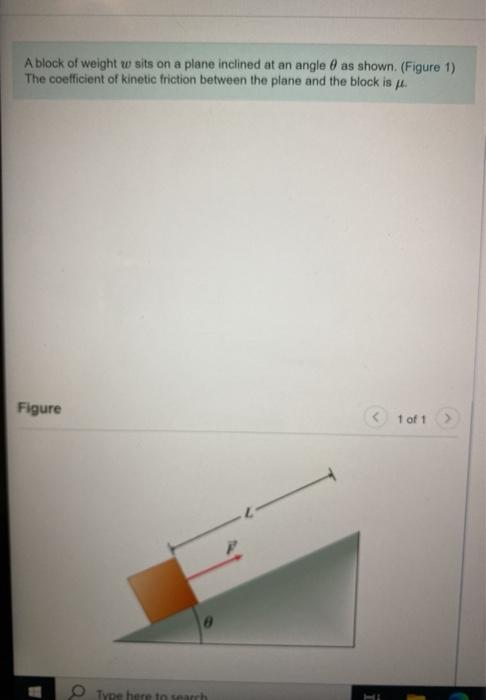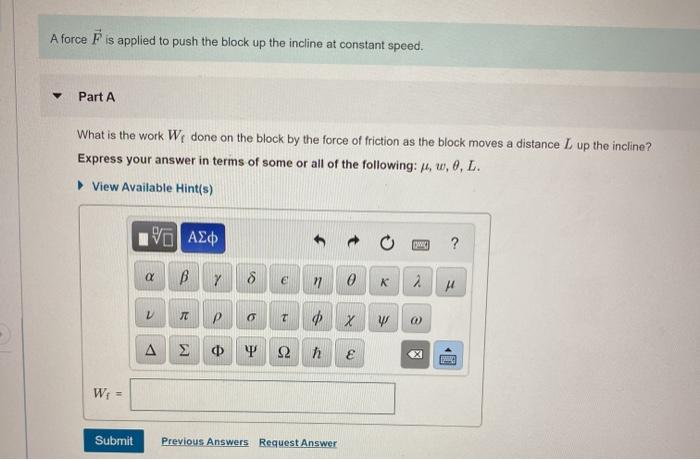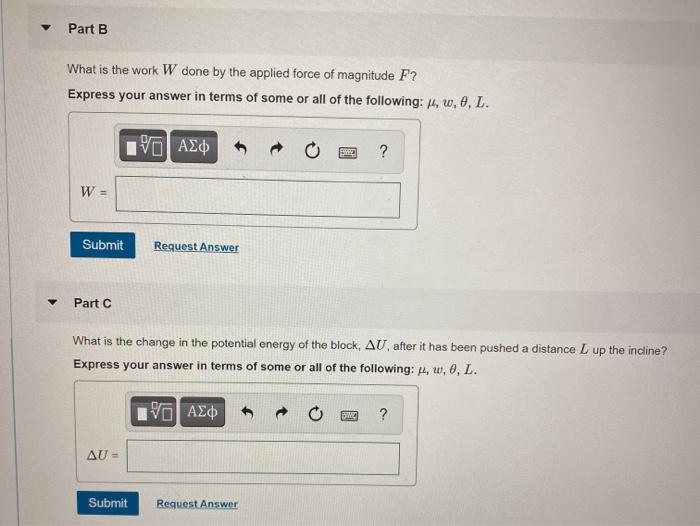# Question A block of weight w sits on a plane inclined at an angle 8 as shown. (Figure 1) The coefficient of kinetic friction between the plane and the block is . Figure 1 of 1 Type here to search A force F is applied to push the block up the incline at constant speed. Part A What is the work W done on the block by the force of friction as the block moves a distance L up the incline? Express your answer in terms of some or all of the following: 4, 0, 0, L. View Available Hint(s) ΨΗ ΑΣΦ ? a B Y 8 e n 0 K 2 TE P T X W 00 Δ Ф т 12 E W = Submit Previous Answers Request Answer Part B What is the work W done by the applied force of magnitude F? Express your answer in terms of some or all of the following: M, w, 8, L. Pal AΣΦ ? W Submit Request Answer Part C What is the change in the potential energy of the block, AU, after it has been pushed a distance L up the incline? Express your answer in terms of some or all of the following: 4, w, 0, L. 18 | Age ? AU= Submit Request Answer